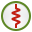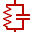# LP Card

This card assigns a parallel circuit of discrete elements to a segment.

On the Source/Load tab, in the Loads / networks group, click theLoad icon. From the drop-down list, click theParallel load (LP) icon.

The figure below depicts the parallel circuit that can be assigned to a segment.

## Parameters:

Define/replace a load at a segment
Define a load with the following parameters.
Remove all LP type loads previously defined
This LP card does not define a load, but rather all previously defined LP loads are deleted. All the other input parameters of this card are ignored.
Reverse feed orientation
By default, the vector of the voltage is orientated in the direction from the start of the segment to its end (the direction in which it was created). When this option is checked, the vector of the voltage is orientated in the opposite direction. (This is the direction of the current flow through the segment. The internal EMF (electromagnetic force) of the impressed voltage source is in the opposite direction.
All segments with this label are assigned the parallel circuit values specified below.
Resistor value (Ohm)
Value of the resistor in $\Omega$
Inductor value (H)
Value of the inductor in H.
Capacitor value (F)
Value of the capacitor in F.

The impedance is then given by

(1) ${Z}_{p}=\frac{1}{\frac{1}{{R}_{p}}+\frac{1}{j\omega {L}_{p}}+j\omega {C}_{p}}$

If the resistance is set to zero, then the resistance is interpreted as infinite, therefore in the parallel case it will not change the impedance. The same applies to the inductance.

The LP card may be combined with the LD, LS, LZ and the SK cards, but only one LP card may be used per label. If a second LP card is used, it replaces the values entered by the first one. This card has no significance for surface elements, even when these are assigned the same label.

Note: Even though the circuit is a parallel circuit, the circuit as a whole is placed in series with the segment to which it is applied.